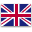UFO Battery is a professional supplier of lithium-ion batteries and power solutions.English
Home > RESOURCES > Knowledge Base > How long will a 12-volt battery run a refrigerator?
Bll Categories
More
Latest Blog
More
Blog Tag
More

# How long will a 12-volt battery run a refrigerator?

by：hqt

In previous article, we know that lithium battery can be used for home backup power, and the fact that refrigerator has an initial spike of starting watts has an influence on the time the battery can last during the outage. When the only load of the 12-volt battery is a refrigerator, this is another history, especially when the refrigerator can be either a residential one at 110-220V or a RV fridge at 12V for camping.

Given that load factor is 80% and the residential and RV refrigerators are mid-sized energy star certified refrigerators in the example, the calculation will be based on the 80% DOD for lithium battery and 50% for the lead-acid battery.

1. For the case of 110-220V residential refrigeratorSince residential refrigerator has an initial spike of starting watts, assuming it is 240 watts for regular running while starting watts is 720 watts, three times of the running power in this example, and thus the inverter capacity should be at least 1500 watts.

If the 12-volt battery is a deep cycle lithium battery, and the volt amperes is equal to 1500/12/80%= 156 amperes which is the smallest capacity provided by the 12 Volt lithium battery. That is, the deep cycle lithium battery is rated at 12 Volt 200Ah. Considering that battery power (Wh)= battery capacity (AH) X battery voltage (V) X number of batteries = 200 X 12 X 1=2400 Watts, then the backup time is calculated as battery power (Wh) / connected load (W) =2400/240=10 hours.

2. For the case of 12V RV refrigeratorConsidering the refrigerator is rated at 150W-400W, the running power is 250W and the starting watts is 750 watts, then the inverter capacity should be at least 1500 watts. If the 12-volt battery is a lead-acid car battery, then the volt amperes is 1500/12/50%= 250 amperes which is the capacity provided by the lead-acid car battery. Then the battery power is 250 X 12 X 1=3000 Watts, then the backup time is 3000/250= 12 hours. If the lead-acid battery is rated at 200Ah, then the power is 200 X 12 X 1=2400 Watts, then the backup time is 2400/250=9.6 hours.

In conclusion, if the battery is rated at 12V 200 Ah, the lithium ion solar battery can power the refrigerator for 10 hours and lead-acid battery for 9.6 hours.

Chat Online 编辑模式下无法使用
Chat Online inputting...